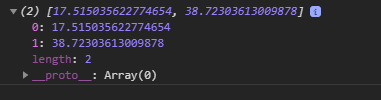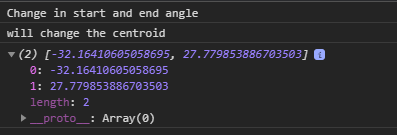# D3.js arc.centroid() Function

• Last Updated : 31 Aug, 2020

The arc.centroid() function is used to compute the midpoint of the centerline of the arc. The midpoint that is generated is calculated using (startAngle+endAngle)/2 and (innerRadius+outerRadius)/2.

Syntax:

Hey geek! The constant emerging technologies in the world of web development always keeps the excitement for this subject through the roof. But before you tackle the big projects, we suggest you start by learning the basics. Kickstart your web development journey by learning JS concepts with our JavaScript Course. Now at it's lowest price ever!

`arc.centroid(arguments);`

Parameters: This function accepts a single parameter as mentioned above and described below.

• arguments: These are the arbitrary arguments.

Return Values: This function return an array containing the points.

Example 1:

## HTML

 ` ``<``html` `lang``=``"en"``> `` ` `<``head``> ``    ``<``meta` `charset``=``"UTF-8"` `/> ``    ``<``meta` `name``=``"viewport"``          ``content="``width``=``device``-width, ``                   ``initial-scale``=``1``.0"/> ``     ` `    ````    ``<``script` `src``= ``        ``"https://d3js.org/d3.v6.min.js"``> ``    ```` `` ` `<``body``>``    ``<``script``> `` ` `        ``// Creating an arc``        ``var arc = d3.arc()``            ``.innerRadius(40)``            ``.outerRadius(45)``            ``.startAngle(10)``            ``.endAngle(8);`` ` `        ``// arc.centroid function ``        ``console.log(arc.centroid());``    `` ````  ` ``

Output:Example 2:

## HTML

 ` ``<``html` `lang``=``"en"``> `` ` `<``head``> ``    ``<``meta` `charset``=``"UTF-8"` `/> ``    ``<``meta` `name``=``"viewport"``          ``content="``width``=``device``-width, ``                  ``initial-scale``=``1``.0"/> `` ` `    ````    ``<``script` `src``= ``        ``"https://d3js.org/d3.v6.min.js"``> ``    ```` `` ` `<``body``>``    ``<``script``> `` ` `        ``// Creating an arc``        ``var arc = d3.arc()``            ``.innerRadius(40)``            ``.outerRadius(45)``            ``.startAngle(0)``            ``.endAngle(8);``        ``// arc.centroid function ``        ``console.log("Change in start and end angle");``        ``console.log("will change the centroid");``        ``console.log(arc.centroid());``    `` ````  ` ``

Output:My Personal Notes arrow_drop_up